# ModelicaRes Tutorial¶

This tutorial shows how to use ModelicaRes to load and analyze Modelica results.

Before we get started, we'll load some standard modules and establish settings for this IPython notebook.

In :
import numpy as np
import matplotlib.pyplot as plt
import pandas; pandas.set_option('display.max_rows', 5)
%matplotlib inline
%precision 4

Out:
u'%.4f'

## Analyzing a simulation result¶

First, we'll load a simulation result of Modelica.Electrical.Analog.Examples.ChuaCircuit:

In :
from modelicares import SimRes
sim = SimRes('ChuaCircuit.mat')


Now we'll explore the simulation results using the methods in SimRes. To get the start and stop times of the simulation, we can look up the initial and final values of time:

In :
sim['Time'].IV()

Out:
0.0
In :
sim['Time'].FV()

Out:
2500.0

There are other many other methods. For example,

In :
Li = sim['L.i']
dict(description=Li.description,
maximum=Li.max(),
mean=Li.mean(),
minimum=Li.min(),
rms=Li.RMS(),
unit=Li.unit)

Out:
{'description': u'Current flowing from pin p to pin n',
'maximum': 3.9185011,
'mean': 0.43196017,
'minimum': -3.3101323,
'rms': 1.699348,
'unit': 'A'}

Notice, however, that description and unit are not methods but fields. To retrieve the values of a variable, use values():

In :
sim['L.v'].values(t=(0,20))

Out:
array([ 0.    ,  0.1092,  0.2108,  0.3046,  0.3904], dtype=float32)

Here we've only included the first 20 seconds, but all of the values are returned by default. To return an array with columns for times and values, use array():

In :
sim['L.v'].array(t=(0,20))

Out:
array([[  0.    ,   0.    ],
[  5.    ,   0.1092],
[ 10.    ,   0.2108],
[ 15.    ,   0.3046],
[ 20.    ,   0.3904]], dtype=float32)

These values have been extracted directly, but it's also possible to interpolate:

In :
sim['L.v'].array(t=[2.5, 7.5, 12.5, 17.5])

Out:
array([[  2.5   ,   0.0546],
[  7.5   ,   0.16  ],
[ 12.5   ,   0.2577],
[ 17.5   ,   0.3475]])

or to return only every nth sample (here, n = 100):

In :
sim['L.v'].array(t=(None,None,100))

Out:
array([[  0.0000e+00,   0.0000e+00],
[  4.8000e+02,   2.3431e-01],
[  9.6000e+02,  -5.2274e-01],
[  1.4400e+03,   1.2706e-01],
[  1.9400e+03,  -1.3850e-01],
[  2.4400e+03,   1.3846e-01]], dtype=float32)

We can perform calculations on the values while retrieving them. For example, to calculate the rate of heat generated by the resistor Ro in the ChuaCircuit at 100 s:

In :
R = sim['Ro.R'].value()
square = lambda x: x**2
Isquared = sim['Ro.i'].values(f=square, t=100)
Isquared*R

Out:
0.1294

As expected, this matches the value recorded in the simulation:

In :
sim['Ro.LossPower'].values(t=100)

Out:
0.1294

To check if a variable is included in the results, we can use Python's in operator.

In :
'L.v' in sim

Out:
True

To see how many variables are in a simulation, use Python's len() function:

In :
len(sim)

Out:
62

To search for variable names, use names() with wildcards:

In :
sim.names('L*v')

Out:
['L.p.v', 'L.v', 'L.n.v']

Regular expressions can also be used:

In :
voltages = sim.names('^[^.]*.v\$', re=True)
voltages

Out:
['G.v', 'L.v', 'C2.v', 'Nr.v', 'Ro.v', 'C1.v']

We can access information about all these variables at once:

In :
sim(voltages).IV()

Out:
[-4.0, 0.0, 0.0, 4.0, 0.0, 4.0]

Notice that we used parentheses; sim[] only accepts one variable and would have produced an error.

We can plot these voltages using plot():

In :
ax, __ = sim.plot(voltages)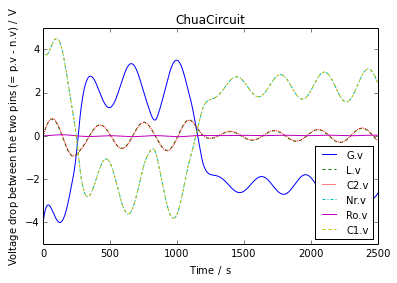The title, axis labels, and legend entries were generated automatically, but they can be customized using other plot arguments or functions from matplotlib.pyplot. The abscissa can be any variable, not just time. Here, we use current:

In :
ax, __ = sim.plot(ynames1='L.v', ylabel1="Voltage", xname='L.i',  xlabel="Current",
title="Chua circuit\n"
"Current through and voltage across the inductor")

# Mark the start and stop points.
def mark(time, text):
i = sim['L.i'].values(time)
v = sim['L.v'].values(time)
plt.plot(i, v, 'bo')
ax.annotate(text, xy=(i, v), xytext=(0, -4), ha='center', va='top',
textcoords='offset points')
mark(0, "Start")
mark(2500, "Stop")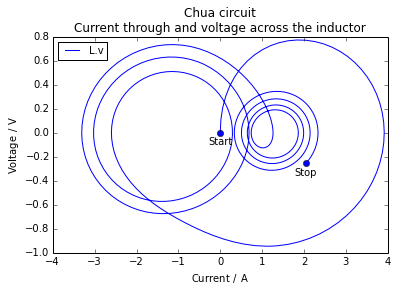In :
ax, __ = sim.plot('L.v', ylabel1="Voltage",
f1={"abs(L.v)": lambda x: np.abs(x)},
title="Chua circuit\nVoltage across the inductor")

# Show the max, mean, and rectified mean.
Lv = sim['L.v']
["max", "mean", "rectified mean"], color='k', linestyle=":")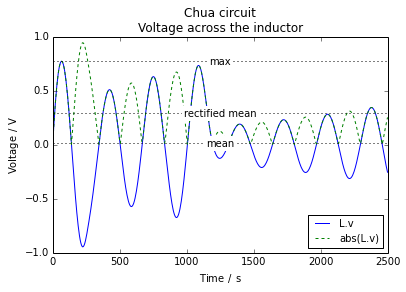We can add traces that are a function of other variables in the plot using the f1 and f2 plot arguments. Here, we will plot the absolute value of the voltage:

We can create a pandas data frame of selected data:

In :
sim.to_pandas(voltages)

Out:
C1.v / V C2.v / V G.v / V L.v / V Nr.v / V Ro.v / V
Time / s
0 4.000000 0.000000 -4.000000 0.000000 4.000000 0.000000
5 3.882738 0.109426 -3.773312 0.109235 3.882738 0.000191
10 3.802946 0.211583 -3.591363 0.210835 3.802946 0.000748
15 3.756024 0.306268 -3.449755 0.304624 3.756024 0.001645
20 3.737386 0.393254 -3.344131 0.390401 3.737386 0.002854
... ... ... ... ... ...

514 rows × 6 columns

The frame has methods to export the results to various formats (e.g., to_clipboard, to_csv, to_excel, to_hdf, to_html, and to_latex).

## Analyzing a linearization result¶

We'll load a linearization result of Modelica.Blocks.Continuous.PID:

In :
from modelicares import LinRes
lin = LinRes('PID.mat')


We can explore it and create diagrams using the methods in LinRes. An instance of LinRes has a sys attribute, which is an instance of the StateSpace class from the control package. The state names are available in sys.state_names:

In :
lin.sys.state_names

Out:
['I.y', 'D.x']

The input and output names are also available:

In :
lin.sys.input_names

Out:
['u']
In :
lin.sys.output_names

Out:
['y']

The system matrixes are available in sys.A, sys.B, sys.C, and sys.D, e.g.,

In :
lin.sys.A

Out:
matrix([[   0.,    0.],
[   0., -100.]])

We can create a Bode plot using bode():

In :
ax1, ax2 = lin.bode(omega=2*np.pi*np.logspace(-2, 3))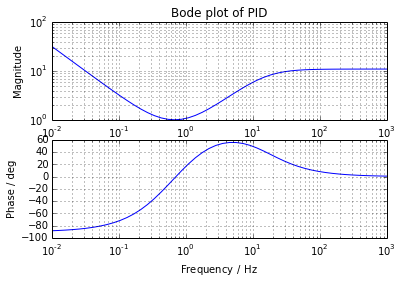Nyquist plots are available via nyquist():

In :
ax = lin.nyquist(omega=2*np.pi*np.logspace(0, 3, 61), labelFreq=20)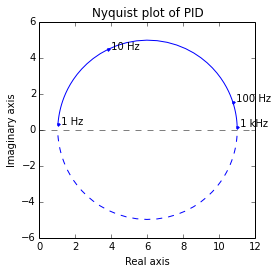## Analyzing groups of results¶

We can also use ModelicaRes to analyze and plot data from multiple simulation and linearization results at once. First, we'll import some additional classes:

In :
from modelicares import SimResList, LinResList


SimResList or LinResList are Python lists with additional methods that operate on the contained simulation and linearization results as a group. Each entry is an instance of SimRes or LinRes (correspondingly).

#### Simulations¶

We'll load all simulations from a nested folder:

In :
sims = SimResList('ChuaCircuit/*/*.mat')
print(sims)

List of simulation results (SimRes instances) from the following files
in the /media/kld/Storage/Documents/Python/ModelicaRes/examples/ChuaCircuit directory:
2/dsres.mat
1/dsres.mat


We can query the list for an attribute of a variable across all of the simulations:

In :
sims['L.v'].RMS()

Out:
[0.20883614, 0.36637381]

We can also see which initial values are different among the simulations:

In :
unique_IVs = sims.unique_IVs()
unique_IVs

Out:
{'L.L': [10.0, 18.0]}

It's easy to plot variables across all of the simulations:

In :
inductance_label = lambda L: 'w/ L.L = {L} H'.format(L=L)
ax, __ = sims.plot('L.v', title="Chua circuit\nwith varying inductance", ylabel1='Voltage',
suffixes=map(inductance_label, unique_IVs['L.L']))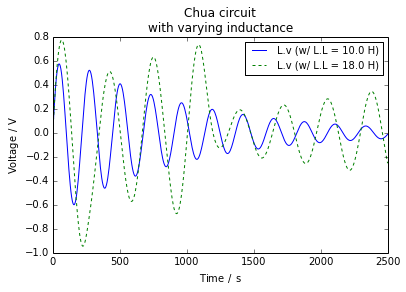#### Linearizations¶

Similarly, we can load two linearizations:

In :
results = {'PID/1/dslin.mat': "Td = 0.1 s",
'PID/2/dslin.mat': "Td = 1 s"}
lins = LinResList(*list(results))


It's necessary to provide the differential time constants because they're not recorded in the files. (However, if each result is accompanied with a "dsin"-style parameter file, we could use read_params(); see the example in LinResList.bode().)

We can create a Bode plot of both linearizations at once:

In :
ax1, ax2 = lins.bode(title="Bode plot of PID\nwith varying differential time constant",
omega=2*np.pi*np.logspace(-2, 3), labels=results.values(),
leg_kwargs=dict(loc='lower right'))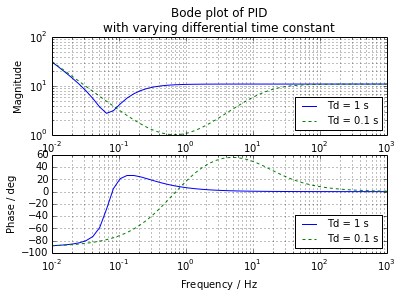or a Nyquist plot:

In :
ax = lins.nyquist(title="Nyquist plot of PID\nwith varying differential time constant",
omega=2*np.pi*np.logspace(-1, 2, 61), labels=results.values(), labelFreq=20)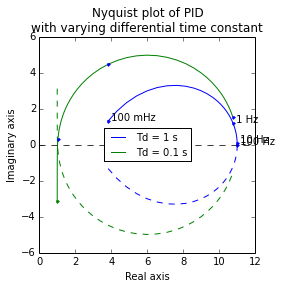## Further information¶

For advanced topics in ModelicaRes, see the the next IPython notebook as a file or a static page. For full documentation of ModelicaRes, see the main webpage.